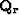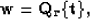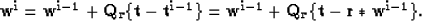Next: Concatenating the two operators Up: SIMULTANEOUS ESTIMATION OF WAVELET Previous: SIMULTANEOUS ESTIMATION OF WAVELET

## Wavelet estimation with a known reflectivity sequence

Although the convolution in equation (1) is commutative, the different spectra ofanddisallow us from using the same operator defined in equation (2) to retrieve the wavelet when the reflectivity sequence is completely known. However, if we start again from the ideal case of a sparse reflectivity sequence, it is possible to define an operatorthat retrieves the exact wavelet:(12)
where the operatoris defined according to(13)
This operator corresponds to a normalized correlation that properly handles edge effects because the norm in the denominator is evaluated over only the active part of r.

A similar approach to the one described for reflectivity estimation can be taken for the wavelet estimation in the case of a non-sparse known reflectivity sequence. The iterative process is then described by(14)Next: Concatenating the two operators Up: SIMULTANEOUS ESTIMATION OF WAVELET Previous: SIMULTANEOUS ESTIMATION OF WAVELET
Stanford Exploration Project
1/13/1998